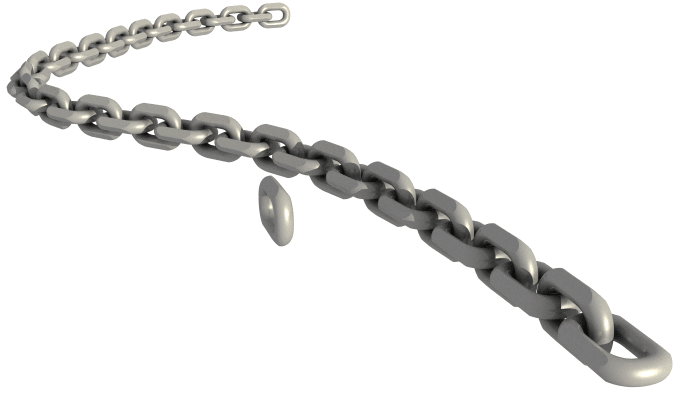# Trying to make a chain

Looking for some tips on doing this correct.

I have a chain link as a closed polysurface and a curve.

I want to array along curve the link and rotate every 2nd link 90ª .

So I can get the object and copy and rotate it. What I am wondering is the array part. I don’t see any commands on the Rhinoscript syntax page.

Here is my script for rotating the object

``````import rhinoscriptsyntax as rs

obj = rs.GetObject("Select object to rotate")

if obj:
bb=rs.BoundingBox(obj)
# find object's bounding box center point
# rotate and copy original object
cplane = rs.WorldXYPlane()
xform = rs.XformRotation2 (90.0, cplane, center)
link2 = rs.TransformObjects( obj, xform, True )

``````

Would it be better to Array along curve first then rotate every second one with …

Slice notation a[low:high:increment]

For now I only have 4 chains to do, so a straight array and then flow along curve works manually for now.

I did see a script about dividing the curve into desired lengths or amounts and then moving object to that.

I will give that a try in a bit.

So I have this so far, but how to make multiple copies.

Here is a test Rhino file …

Chain test-1.3dm (209.8 KB)

``````import rhinoscriptsyntax as rs

crv1 = rs.GetObject("Select a curve")
if rs.IsCurve(crv1):
length = 2.18
points = rs.DivideCurveLength(crv1, length)

obj = rs.GetObject("Select object to copy")
if obj:
start = rs.GetPoint("Point to copy from")
if start:
end = rs.GetPoint("Point to copy to", start)
if end:
translation = end-start
rs.CopyObject( obj, translation )

'''
obj = rs.GetObject("Select object to rotate")

if obj:
bb=rs.BoundingBox(obj)
# find object's bounding box center point
# rotate and copy original object
cplane = rs.WorldXYPlane()
xform = rs.XformRotation2 (90.0, cplane, center)
link2 = rs.TransformObjects( obj, xform, True )

'''``````

Hi Randy,

I edited your script to make it work for all points on the division. I hope the comments are clear else ask for it.``````import rhinoscriptsyntax as rs

crv1 = rs.GetObject("Select a curve")
if rs.IsCurve(crv1):
length = 2.18
#points = rs.DivideCurveLength(crv1, length)
# get parameters on the curve to later use to get the frame on the curve
parameters = rs.DivideCurveLength(crv1, length,return_points=False)

obj = rs.GetObject("Select object to copy")

#set plane to copy from
startplane = rs.WorldYZPlane() #plane perpendicular or link orientation on curve

bbox = rs.BoundingBox(obj)
bbox_center = rs.PointDivide(rs.PointAdd(bbox,bbox),2)# get boundingbox center
#
startplane.Origin = bbox_center #set startplane origin to center
#Links will be placed on the center of the division points

N = -1 # counter to check if we are at even link (even links are rotated)
#matrix to rotate around length axis 90deg
Xrotate = rs.XformRotation2(90,startplane.ZAxis,startplane.Origin)

#for each parameter (point on curve) transform the link to the point on the curve
for parameter in parameters:
N+=1 #add 1 to object counter

if (N % 2 == 0): #even number so we first rotate

# get plane perpendicular on curve at parameter
curve_frame = rs.CurvePerpFrame(crv1,parameter)
Xoncurve = rs.XformRotation1(startplane,curve_frame)
I need a week just to do python